Courses

# Chapter 2 - Mathematical Models Of Physical System - Notes, Control System, Electrical Engineering Electrical Engineering (EE) Notes | EduRev

## Electrical Engineering (EE) : Chapter 2 - Mathematical Models Of Physical System - Notes, Control System, Electrical Engineering Electrical Engineering (EE) Notes | EduRev

The document Chapter 2 - Mathematical Models Of Physical System - Notes, Control System, Electrical Engineering Electrical Engineering (EE) Notes | EduRev is a part of the Electrical Engineering (EE) Course Electrical Engineering SSC JE (Technical).
All you need of Electrical Engineering (EE) at this link: Electrical Engineering (EE)

## TRANSFER FUNCTION

• For nay control systems there exists an input termed as excitation or cause (denoted as r) which operates through a transfer operation termed as transfer function and produces an effect resulting in output or response termed as control variable. Thus the cause and effect relationship between the output and input is related to each other through a transfer function
• The relationship between output and input is represented by a diagram known as block diagram.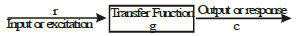• The arrow in the diagram indicates the direction of control action, thus the block diagram has unilateral property in the direction of arrow.
• With, reference to control system, the transfer function is expressed as a ratio of Laplace transform of output to Laplace transform of input.  Thus if

R(s)→ Laplace transform of the input function
C(s)→ Laplace transform of the output function
Then the block diagram will become as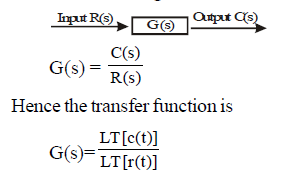• The transfer function of a control system is defined as the ratio of the Laplace transform of the output variable to the laplace transform of the input variable assuming all initial conditions are zero."

METHODS OF ANALYSIS
There are two methods for the analysis of a system.

• Transfer function approach
• State variable approach

• It gives simple mathematical algebraic equation.
• It gives poles and zeros of the system directly.
• Stability of the system can be determined easily
• The output of the system for any input can be determined easily.

It is applicable only for linear time- invariant system.

• It does not take initial conditions into account.
• The internal states of the system cannot be determined.
• Applicable only for single input and single output.
• Controllability and observability cannot be determined.

Control Loop Analysis
There are two basic control loop configurations:
(a) Closed Loop Control System

• In this configuration the changes in the output are measured through feedback and compared with input to achieve the control objective. Consider the negative closed loop system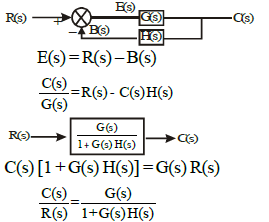(b) Open Loop Control System

• In this configuration feedback is disconnected. No performance analysis is required for open loop control systems.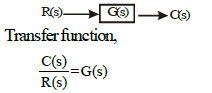Block Diagram and Block Diagram Algebra

• To draw a block diagram for a system, first write the equations that describe the dynamic behaviour of each component. Then take the Laplace transform of these equations, assuming zero initial conditions, and represent each Laplace transformed equations individually in block form. Finally, assemble the elements into a complete block diagram.

Methods of Analysis
Methods of analysis of a system: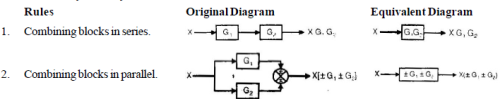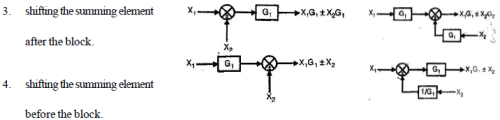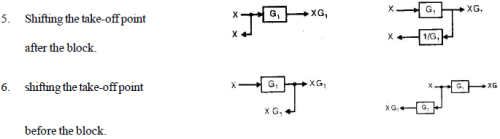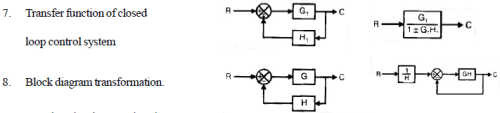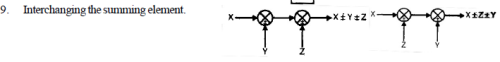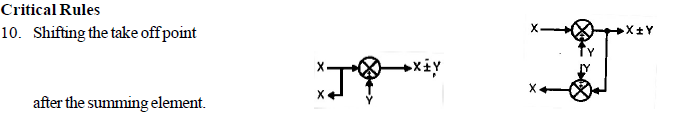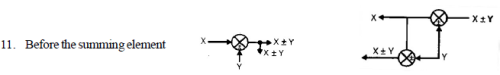SIGNAL FLOW GRAPHS

• it is a graphical representation of set of linear algebraic expressions describing a control system.
• It is a network in which nodes representing each of the system variables are connected by direct branches.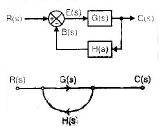Terminology of signal Flow Graphs

• Node: It represents a system variable and is equal to the sum of all incoming signals at it. Input node/Source node: It is node having only outgoing branches. Output node/sink node: It is a node having only incoming branches.
• Path: It is the traversal of the connected branches in the direction of branch arrow. such that no node is traversed more than once.
• Forward path: It is a path from input node to output node (travelling each node once.)
• Loop: It is a path which originates and teminates at the same node:
• Non-touching Loops: Two or more loops are said to be non-touching if they do not have a common node
• Example: Draw the signal flow graph of the control system shown below: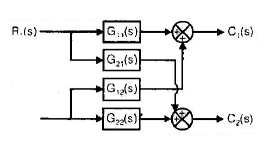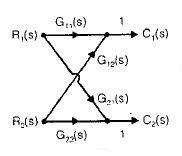Mason's Gain Formula

• The overall gain or transfer function of a control system is given by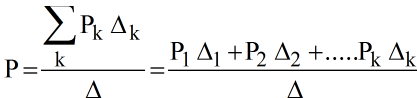where, pk = path gain of kth forward path

D = 1 – (sum of gains of all individual loops) + (sum of gain products of two non-touching loops) – (sum  of gain products of 3 nontouching loops) + ....

Dk = (It is that value of D obtained by removing all the loops touching the kth forward path).

Offer running on EduRev: Apply code STAYHOME200 to get INR 200 off on our premium plan EduRev Infinity!

65 docs|37 tests

,

,

,

,

,

,

,

,

,

,

,

,

,

,

,

,

,

,

,

,

,

,

,

,

,

,

,

;